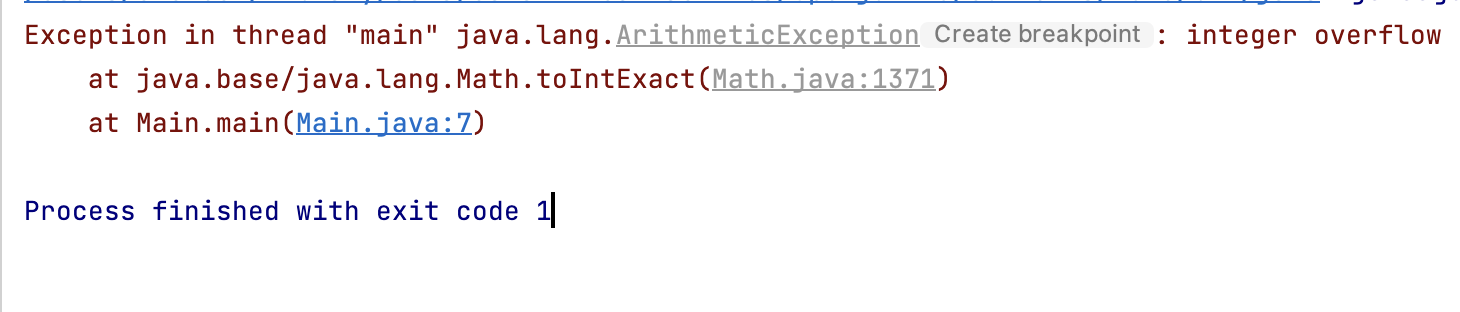# Best way to Convert Primitive long to int in Java with some Cautions

Java is called a hybrid-programming language or not a truly object-oriented language as it supports primitives. Working with primitives may not be easy as it may seem as incorrect type conversions can create havoc!

In Java, when we convert a larger data type such as a double, long to a smaller one such as an int, short, byte, char this process is called "narrowing primitive conversion" which this can result in loss of precision.

Let us take a look at a few ways to convert a Java int type to a primitive long.

## Example 1: By Type-casting to int (not recommended)

Code:
``````    public static void main(String[] args) {
long myLong = 20L;
int myInt = (int) myLong; //typecasting
System.out.println(myInt);
}``````

Output: 20

This may look good, but there is a serious problem that can arise here. int in Java are of 4-bytes and it can hold values between the range -2147483648 to 2147483647, whereas long have a size of 8-bytes so they can hold a signed value of range -9223372036854775808 to 9223372036854775807

So let's try the above code with a value of long as 922337203685.

``````    public static void main(String[] args) {
long myLong = 922337203685L;
int myInt = (int) myLong;
System.out.println(myInt);
}``````
Output:

-1080764955

Note: There was no complication error when I ran this code, but the value int printed is not the same as what I was expecting. This is because we trying to save a value that is over the limit of int causing an integer overflow.

## Example 2: Using toIntExact() method from java.lang.Math package.

toIntExact() method was introduced in Java 8, this is the proper way of typecasting a long to long to int.

Code:
``````import static java.lang.Math.toIntExact;

public class Main {

public static void main(String[] args) {
long myLong = 922337203685L;
int myInt = toIntExact(myLong);
System.out.println(myInt);
}
}``````
Output:
``````Exception in thread "main" java.lang.ArithmeticException: integer overflow
at java.base/java.lang.Math.toIntExact(Math.java:1371)
at Main.main(Main.java:7)``````-

Have Questions? Post them here!